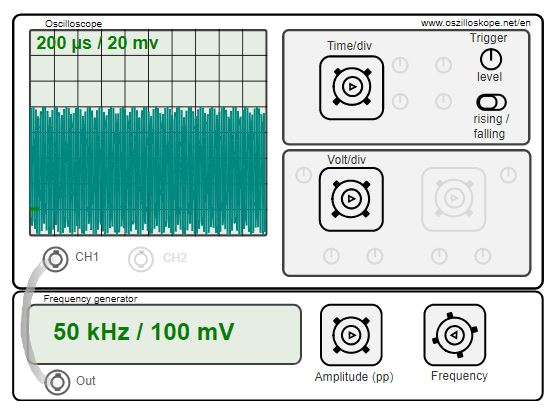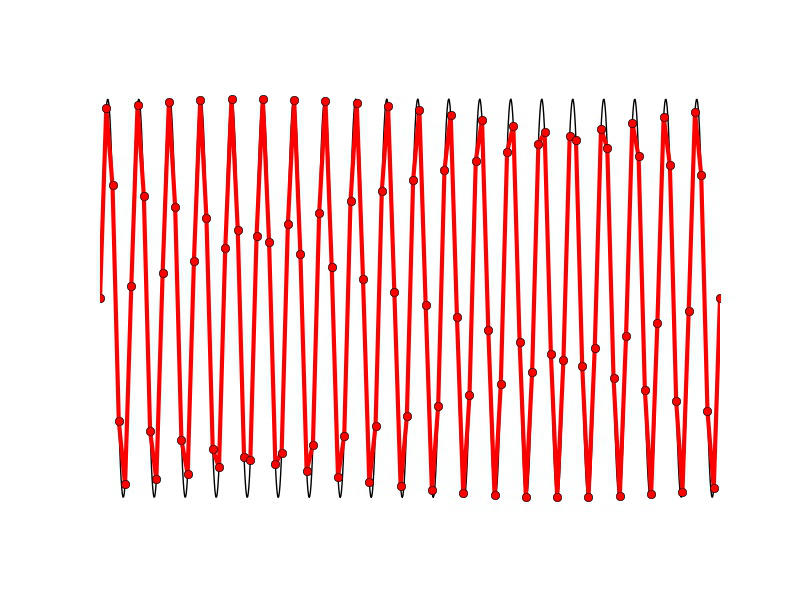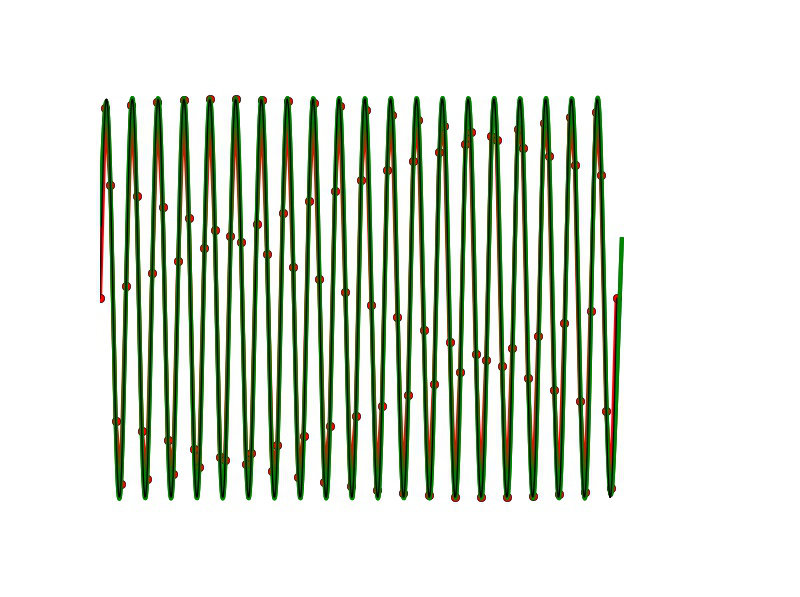Oscilloscope simulator - artifacts occur due to discrete sampling at high frequencies. The signal is sampled internally with 100kHz.

With the simulator one can observe an effect at high frequencies, which leads to an incorrect display of the measurement signal. The signal of 20kHz is sampled internally at approx. 100kHz. The sampling frequency of 100 kHz lies according to the sampling theorem clearly above the signal frequency.

## Signal Reconstruction

The sampling theorem says that the sampling frequency has to be at least a factor two above the highest frequency of the measurement signal. In an oscilloscope, a low-pass filter is used to filter out frequencies exceeding half the sampling frequency.

Although the sampling theorem is adhered to here, the signal is not correctly reconstructed. This is due to the simplified reconstruction by connecting the sampled values with lines. If the frequency of the measurement signal is close to half the sampling frequency, the the sinusoidal signal is only sampled with a few points:Sampling of the measurement signal with 6x sample rate. Simple reconstruction by lines.

The figure above shows the effect. Per sine period (black line) the signal is scanned with 6 points. If you connect the sampled points with lines, one gets different points in each case compared to the sine signal (red line). The result is an amplitude modulation and the black signal is not correctly represented by the red lines. The reason for this is that a simple connection is created by lines does not represent a correct reconstruction. For a high number of sampling points, this approximation is acceptable. For a sampling frequency near the Nyquist-Shannon-Limit this results in problems.Abtastung mit 6facher Samplefrequenz. Rekonstruktion durch Resampling. Sampling at 6x sample rate. Reconstruction by resampling. This corresponds to mathematical zero padding using a window function.

A remedy here is an improved signal reconstruction, e.g. by "Zeropadding". Here, the measured data is replaced by a Fourier transformation into frequency space, zeros are attached and the resulting signal is Fourier transformed back to time space. You get an interpolated signal that reproduces the sine significantly better. In addition, a Window function suppresses border effects. The Nyquist Shannon Limit (sampling frequency>2*signal frequency) strictly speaking only applies to infinitely long signal sequences. With an oscilloscope, you want to have a finite measure time, therefore a window function helps to surpress effects by short measurement intervals. The Fourier transformation requires periodic signals, this is guaranteed by windowing the signal. In the above illustration, the green signal reconstructs the original sinus is much better than the red signal. The oscilloscope simulator uses only a simple reconstruction by lines in advance for a lower computation time.